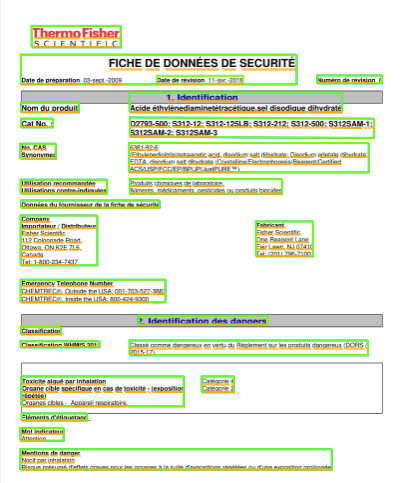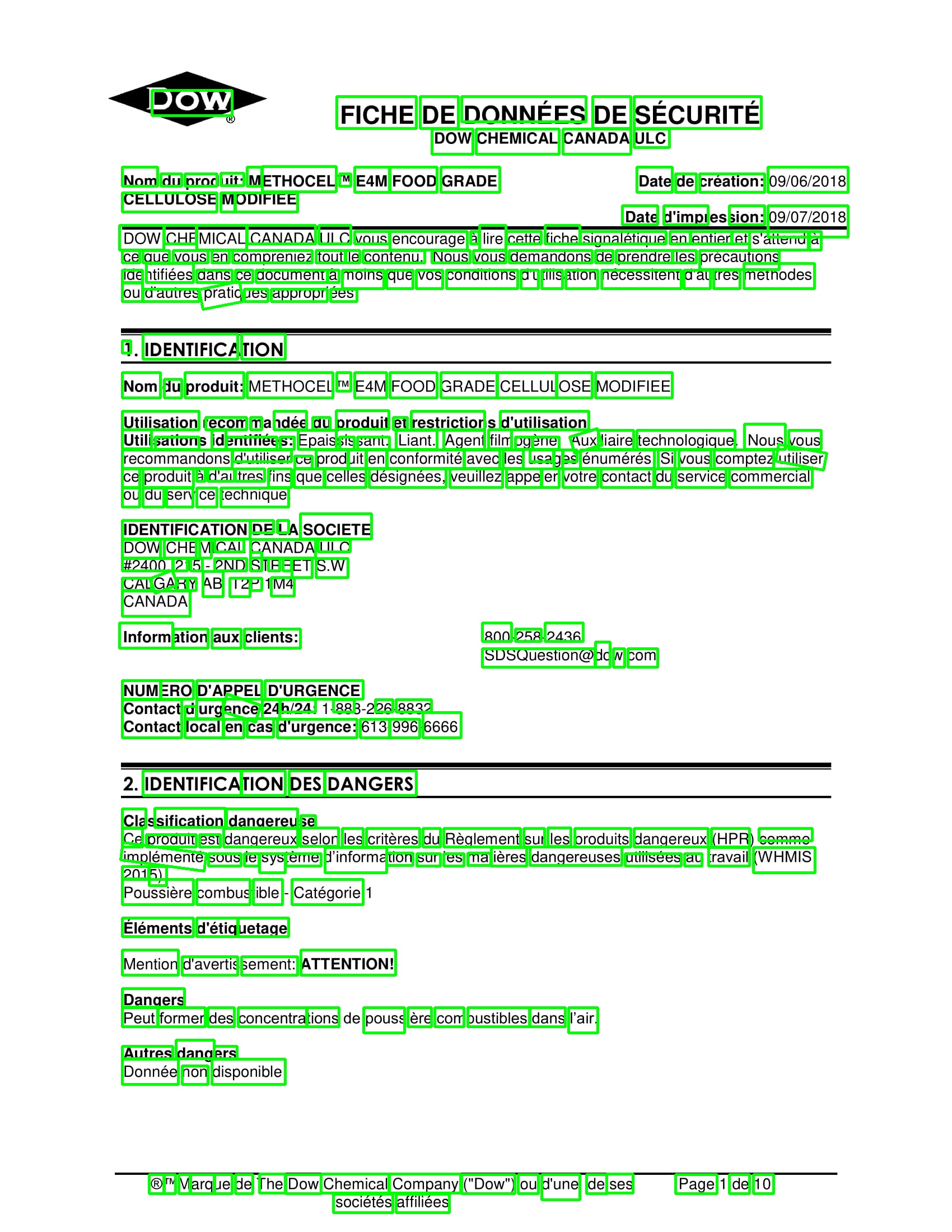#StackBounty: #swift #machine-learning #swift-playground #coreml #createml Evaluation Accuracy is Different When Using Split Table Vers…

Bounty: 100

I am creating a tabular classification model using CreateML and Swift. The dataset I am using has about 300 total items, and about 13 different features. I have tried training/testing my model in two ways and have had surprisingly very different outcomes:

1) Splitting my training and evaluation data table randomly from the original full data set:

``````let (classifierEvaluationTable, classifierTrainingTable) = classifierTable.randomSplit(by: 0.1, seed: 4)
``````

I have played around a bit with the .1 split number and 4 seed number but the results are all over the place: Could be 33% or 80% evaluation accuracy in some cases. (I got 78% training accuracy, 83% validation accuracy, 75% evaluation accuracy in this case.)

2) I manually took 10 items from the original data set and put them into a new data set to test later. I then removed these items from the 300 item data set which was used for training. When I tested these 10 items, I got 96% evaluation accuracy. (I got 98% training accuracy, 71% validation accuracy, 96% evaluation accuracy in this case.)

I am wondering why is there such a big difference? Which reading should be seen as more realistic and credible? Is there anything I can do to either model to improve accuracy and credibility? ALSO: I am confused as to what the different accuracy measurements mean and how I should interpret them (training, validation, evaluation)?

Thanks.

Get this bounty!!!

#StackBounty: #machine-learning #deep-learning #generative-models #gan How to interpret the following GAN training losses?

Bounty: 50

I am training a GAN using the following loss functions:

``````_, d_real_logit = discriminator(x_d)
_, d_fake_logit = discriminator(generator(z_g))
loss_d_real = tf.reduce_mean(tf.nn.sigmoid_cross_entropy_with_logits(labels=tf.ones_like(d_real_logit), logits=d_real_logit))
loss_d_fake = tf.reduce_mean(tf.nn.sigmoid_cross_entropy_with_logits(labels=tf.zeros_like(d_fake_logit), logits=d_fake_logit))
loss_d = loss_d_fake + loss_d_real

_, g_logit = discriminator(generator(z_g))
loss_g = tf.reduce_mean(tf.nn.sigmoid_cross_entropy_with_logits(labels = tf.ones_like(g_logit), logits=g_logit))
``````

where discriminator is defined as:

``````def discriminator(x):
y1_d = tf.nn.leaky_relu(tf.matmul(x, w_d_1) + b_d_1)
y2_d = tf.nn.leaky_relu(tf.matmul(y1_d, w_d_2) + b_d_2)
y3_d = tf.nn.leaky_relu(tf.matmul(y2_d, w_d_3) + b_d_3)
y4_d = tf.nn.leaky_relu(tf.matmul(y3_d, w_d_4) + b_d_4)
logits = tf.matmul(y4_d, w_d_5) + b_d_5
y5_d = tf.nn.sigmoid(logits)

return y5_d, logits
``````

The plots of loss functions obtained are as follows:I understand that g_loss = 0.69 and d_loss = 1.38 are ideal situations, since that corresponds to discriminator output being 0.5 for both real and fake samples.
But, for some reason the 2 loss values move away from these desired values as the training goes on. Does anyone know why this happens?
(x axis is number of epochs/100)

Get this bounty!!!

#StackBounty: #machine-learning #bayesian #model #train #online Practical realities of updating a trained model with new data

Bounty: 50

In my day to day work, I train models on data using R packages that have no extension for Bayesian priors. I will generally have a large dataset to start off with, and add new data as needed.

Any time I want to update the model, I have to train the entire thing from scratch.

Are there ways of mitigating the considerable and slowly-increasing time cost of re-training everything from scratch, when I am unable to use Bayesian priors in my model?

A couple of approaches have occurred to me. Model training generally allows for initial weights/parameters to be specified. Setting the initial weights to the weights of the previous model may be a start, but presumably you need to include the previous data, or else the model will move from the old weights to capture only the new data.

Does training old + new data using initial weights trained from old data decrease the training time appreciably? Are there any other practical considerations for dealing with this type of situation?

Get this bounty!!!

#StackBounty: #machine-learning #cnn #computer-vision How to detect blocks of texts in document images

Bounty: 50

I am planning to detect texts from document text images like below:

GOAL:WORK DONE:
I have tried to solve this with some scene text detection algorithms like EAST Text detector and PixelLink. But it only provides result in such a way it detects each and every word individually as below, which is obvious:What method can help me detect blocks of texts as mentioned under GOAL.

EDIT :

I don’t want extract all texts via OCR. What I want instead is to detect texts based on their visual positional arrangement. See in the image, texts positioned together are detected as blocks. And my result should contain all the bounding box co-ordinates of all the detected text blocks.

Get this bounty!!!

#StackBounty: #machine-learning #classification What is the definition of margin for multi-class classification?

Bounty: 50

I heard the definition was as follows:

Let \$y_{best} = arg max_{c in Classes} f(x)_c\$ be the best class and let the prediction function be an output vector \$f(x) in R^{|Classes|}\$. Then define:

\$\$ margin = f(x){y{best}} – max_{c neq y_{best}} f(x)_c\$\$

there seems something fishy becaue for me there should be some sense of dividing by some “normalization” because otherwise it seems like “functional margin”. Anyway, is this correct? How does it compare to the binary margin and functional margin definitions?

Get this bounty!!!

#StackBounty: #machine-learning #dataset #computer-vision #tensorflow How to make predictions on test image after training inception mo…

Bounty: 50

I just finished training inceptionv3 from scratch on my custom dataset(1675 train images, 400 validation images, 2 classes):

1. I don’t know how to make predictions on my test images using my newly trained model.(where to point label_image.py for model)

2. Where did my newly trained model got saved?

3. I got following files generated in train_dir:

• events.out.tfevents.1481980070.airig-Inspiron-7559(4.9GB)
• graph.pbtxt(18.5MB)
• and a bunch of model.ckpt-.meta and model.ckpt-.index files

After running train script I got:-

``````....
INFO:tensorflow:Stopping Training.
INFO:tensorflow:Finished training! Saving model to disk.
``````

After running eval script I got:–

``````.....
INFO:tensorflow:Evaluation [0/25]
INFO:tensorflow:Evaluation [1/25]
INFO:tensorflow:Evaluation [2/25]
INFO:tensorflow:Evaluation [3/25]
INFO:tensorflow:Evaluation [5/25]
INFO:tensorflow:Evaluation [5/25]
INFO:tensorflow:Evaluation [6/25]
INFO:tensorflow:Evaluation [7/25]
INFO:tensorflow:Evaluation [8/25]
INFO:tensorflow:Evaluation [9/25]
INFO:tensorflow:Evaluation [10/25]
INFO:tensorflow:Evaluation [11/25]
INFO:tensorflow:Evaluation [13/25]
INFO:tensorflow:Evaluation [13/25]
INFO:tensorflow:Evaluation [14/25]
INFO:tensorflow:Evaluation [15/25]
INFO:tensorflow:Evaluation [16/25]
INFO:tensorflow:Evaluation [17/25]
INFO:tensorflow:Evaluation [18/25]
INFO:tensorflow:Evaluation [19/25]
INFO:tensorflow:Evaluation [20/25]
INFO:tensorflow:Evaluation [21/25]
INFO:tensorflow:Evaluation [22/25]
INFO:tensorflow:Evaluation [23/25]
INFO:tensorflow:Evaluation [25/25]
I tensorflow/core/kernels/logging_ops.cc:79] eval/Recall@5
I tensorflow/core/kernels/logging_ops.cc:79] eval/Accuracy
INFO:tensorflow:Finished evaluation at 2016-12-19-03:59:04
``````

Get this bounty!!!

#StackBounty: #machine-learning #image-processing Object Localisation without Classification

Bounty: 50

I have a data set of photos containing an object in each of them. I want to find out the coordinates of rectangle enclosing the object.

Note that each photo contains exactly 1 object (for example, if there is a pair of shoes in the photo it is to be treated as one object), and the photos are taken in a simple white background. But the images do not contain one class of objects, the object can be anything.

I have a training set, consisting of photos, and the coordinates of the rectangle enclosing the object for these photos. And I want to find the coordinates of the enclosing rectangle, given a new photo (exactly 1 object, photos taken in simple white background).

I searched a lot for a method to do so, and found resources for achieving localization with classification, but neither do I want to classify the objects nor do I have class labels in my training set.

I also thought edge detection and object segmentation methods could be useful.

However, I feel that my task is much simpler since I know that I have to localize only 1 object in an image and the background is also simple, so there must be some simple methods I am overlooking.

Any guidance is much appreciated, and I am relatively new to machine learning so I would be grateful for guidance to implement the appropriate technique.

Get this bounty!!!

#StackBounty: #machine-learning #definition Why is the risk equal to the emperical risk when taking the expectation over the samples?

Bounty: 50

From Understanding Machine Learning: From theory to algorithms:

Let $$S$$ be a set of $$m$$ samples from a set $$Z$$ and $$w^*$$ be an arbitrary vector. Then $$Bbb E_{S text{ ~ } D^m}[L_S(w^*)] = L_D(w^*)$$.

Where: $$L_S(w^*) equiv frac{1}{m}sum_{i=1}^ml(w^*, z_i)$$ and $$z_i in S$$, $$L_D(w^*) equiv Bbb E_{z text{ ~ }D}[l(w^*, z)]$$, $$D$$ is a distribution on $$Z$$, and $$l(text{_},text{_} )$$ is a loss function.

I see that $$Bbb E_S[L_S(w^)] = Bbb E_S[frac{1}{m}sum_{i=1}^ml(w^, z_i)] = frac{1}{m}sum_{i=1}^m Bbb E_S[l(w^*, z_i)]$$ and
$$L_D(w^) = Bbb E_z[l(w^, z)] = sum_{z in Z} l(w^*, z)D(z)$$

But how are these two equal? $$Bbb E_S$$ is an expectation over samples $$S$$ of size $$m$$ whereas $$Bbb E_z$$ is an expectation over all samples in $$Z$$.

Get this bounty!!!

#HackerRank: Computing the Correlation

Problem

You are given the scores of N students in three different subjects – MathematicsPhysics and Chemistry; all of which have been graded on a scale of 0 to 100. Your task is to compute the Pearson product-moment correlation coefficient between the scores of different pairs of subjects (Mathematics and Physics, Physics and Chemistry, Mathematics and Chemistry) based on this data. This data is based on the records of the CBSE K-12 Examination – a national school leaving examination in India, for the year 2013.

Pearson product-moment correlation coefficient

This is a measure of linear correlation described well on this Wikipedia page. The formula, in brief, is given by:where x and y denote the two vectors between which the correlation is to be measured.

Input Format

The first row contains an integer N.
This is followed by N rows containing three tab-space (‘\t’) separated integers, M P C corresponding to a candidate’s scores in Mathematics, Physics and Chemistry respectively.
Each row corresponds to the scores attained by a unique candidate in these three subjects.

Input Constraints

1 <= N <= 5 x 105
0 <= M, P, C <= 100

Output Format

The output should contain three lines, with correlation coefficients computed
and rounded off correct to exactly 2 decimal places.
The first line should contain the correlation coefficient between Mathematics and Physics scores.
The second line should contain the correlation coefficient between Physics and Chemistry scores.
The third line should contain the correlation coefficient between Chemistry and Mathematics scores.

So, your output should look like this (these values are only for explanatory purposes):

``````0.12
0.13
0.95
``````

Test Cases

There is one sample test case with scores obtained in Mathematics, Physics and Chemistry by 20 students. The hidden test case contains the scores obtained by all the candidates who appeared for the examination and took all three tests (Mathematics, Physics and Chemistry).
Think: How can you efficiently compute the correlation coefficients within the given time constraints, while handling the scores of nearly 400k students?

Sample Input

``````20
73  72  76
48  67  76
95  92  95
95  95  96
33  59  79
47  58  74
98  95  97
91  94  97
95  84  90
93  83  90
70  70  78
85  79  91
33  67  76
47  73  90
95  87  95
84  86  95
43  63  75
95  92  100
54  80  87
72  76  90
``````

Sample Output

``````0.89
0.92
0.81
``````

There is no special library support available for this challenge.